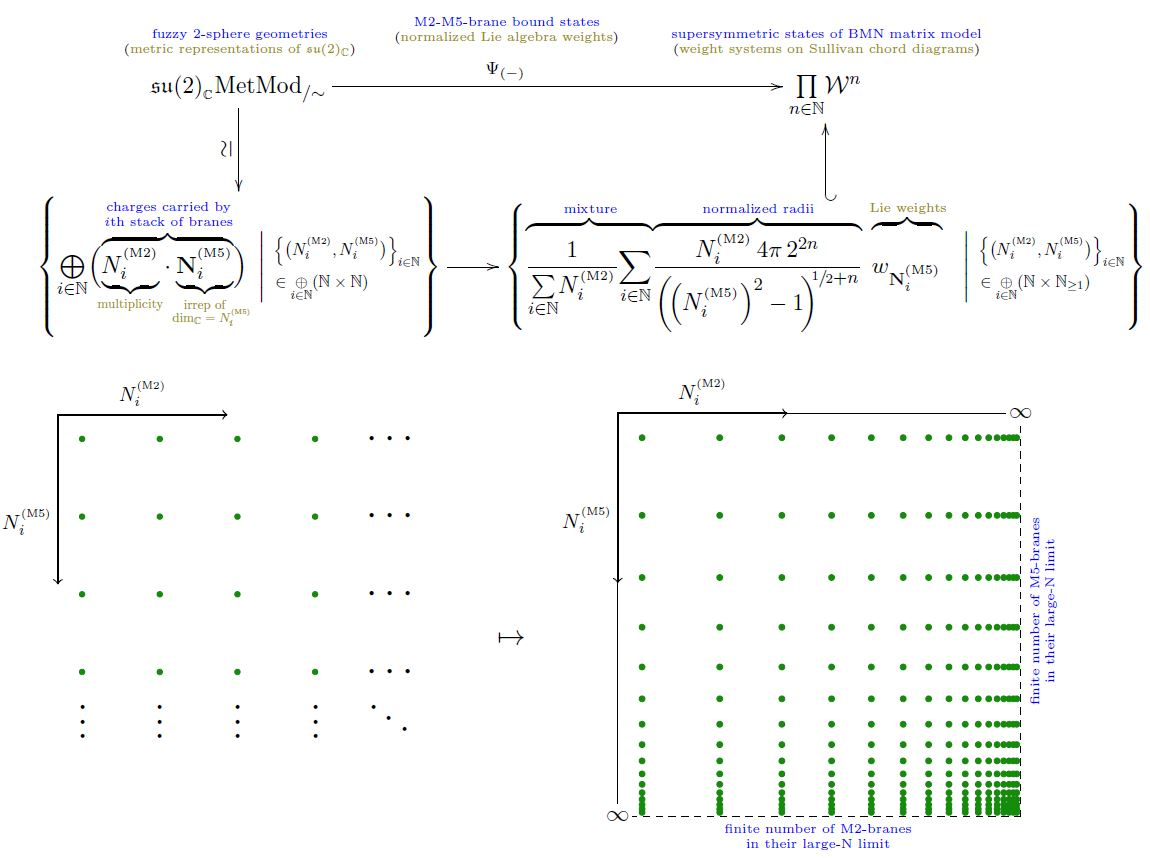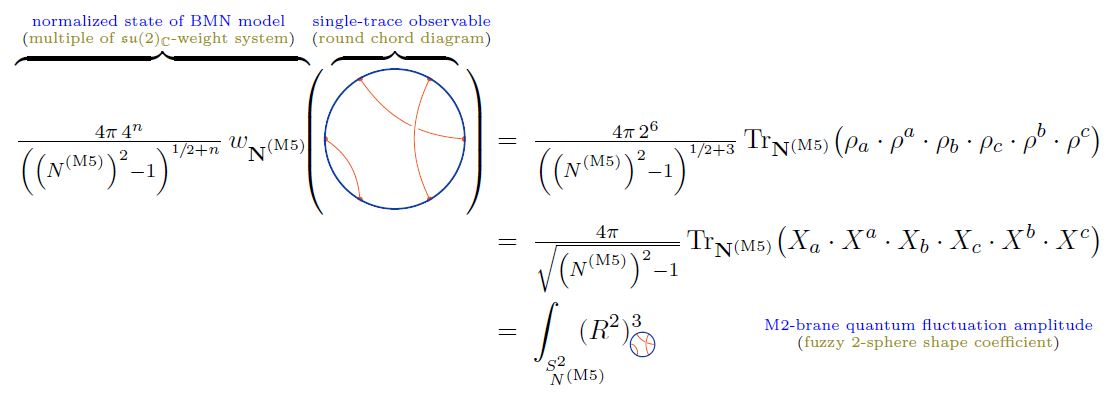Contents

# Contents

## Idea

in particular dyonic$\,$black M2-branes, i.e. M5-branes wrapped on a 3-manifold (ILPT 95)

## Properties

### Image in type II string theory

Under suitable duality between M-theory and type IIA string theory the M2/M5brane bound states become Dp-D(p+2)-brane bound states (Basu-Harvey 05).

### Relation to giant gravitons

for the relation to giant gravitons: (Camino-Ramallo 01)

### M2-M5 brane bound states in the BMN matrix model

There is the suggestion (MSJVR 02, checked in AIST 17a, AIST 17b) that, in the BMN matrix model, supersymmetric M2-M5-brane bound states are identified with isomorphism classes of certain “limit sequences” of longitudinal-light cone-constant $N \times N$-matrix-fields constituting finite-dimensional complex Lie algebra representations of su(2).

Concretely, if

$\underset{ i }{\oplus} \big( N^{(M2)}_i \cdot \mathbf{N}^{(M5)}_i \big) \;\;\in\;\; \mathfrak{su}(2)_{\mathbb{C}}Rep^{fin}$

denotes the representation containing

of the

• $N^{(M5)}_i$-dimensional irrep $\mathbf{N}^{(M5)}_i \in \mathfrak{su}(2)_{\mathbb{C}}Rep$

(for $\{N^{(M2)}_i, N^{(M5)}_i\}_{i} \in (\mathbb{N} \times \mathbb{N})^I$ some finitely indexed set of pairs of natural numbers)

with total dimension

$N \;\coloneqq\; dim \big( \underset{ i }{\oplus} \big( N^{(M2)}_i \cdot \mathbf{N}^{(M5)}_i \big) \big)$

then:

1. a configuration of a finite number of stacks of coincident M5-branes corresponds to a sequence of such representations for which

1. $N^{(M2)}_i \to \infty$ (this being the relevant large N limit)

2. for fixed $N^{(M5)}_i$ (being the number of M5-branes in the $i$th stack)

3. and fixed ratios $N^{(M2)}_i/N$ (being the charge/light-cone momentum carried by the $i$th stack);

2. an M2-brane configuration corresponds to a sequence of such representations for which

1. $N^{(M5)}_i \to \infty$ (this being the relevant large N limit)

2. for fixed $N^{(M2)}_i$ (being the number of M2-brane in the $i$th stack)

3. and fixed ratios $N^{(M5)}_i/N$ (being the charge/light-cone momentum carried by the $i$th stack)

for all $i \in I$.

Hence, by extension, any other sequence of finite-dimensional $\mathfrak{su}(2)$-representations is a kind of mixture of these two cases, interpreted as an M2-M5 brane bound state of sorts.

#### Formalization via weight systems on chord diagrams

To make this precise, let

$\mathfrak{su}(2)_{\mathbb{C}} MetMod_{/\sim} \;\in\; Set$

be the set of isomorphism classes of complex metric Lie representations (hence finite-dimensional representations) of su(2) (hence of the special linear Lie algebra $\mathfrak{sl}(2,C)$) and write

$Span \big( \mathfrak{su}(2)_{\mathbb{C}} MetMod_{/\sim} \big) \;\in\; Vect_{\mathbb{C}}$

Finally, write

$\array{ Span \big( \mathfrak{su}(2)_{\mathbb{C}}MetMod_{/\sim} \big) & \longrightarrow & \big( \mathcal{W}^{{}^{s}} \big)^{deg} \\ c_1 \cdot V_1 + c_2 \cdot V_2 &\mapsto& c_1 \cdot w_{V_1} + c_2 \cdot w_{V_2} }$

for the linear map which sends a formal linear combination or representations to the weight system on Sullivan chord diagrams with $deg \in \mathbb{N}$ chords which is given by tracing in the given representation.

Then a M2-M5-brane bound state as in the traditional discussion above, but now formalized as an su(2)-weight system

$\Psi_{ \left\{ N^{(M2)}_i, N^{(M5)}_i \right\}_{i} } \;\in\; \underset{ deg \in \mathbb{N} }{\prod} \big( \mathcal{W}^{{}^{\mathrm{s}}} \big)^{deg}$

hence a weight system horizontal chord diagrams closed to Sullivan chord diagrams, these now being the multi-trace observables on these) is

(1)$\Psi_{ \left\{ N^{(M2)}_i, N^{(M5)}_i \right\}_{i} } \;\coloneqq\; \tfrac{ 4 \pi \, 2^{2\,deg} }{ \sum_i N^{(M2)}_i } \underset{i}{\sum} \left( N^{(M2)}_i \cdot \tfrac{1}{ \left( \sqrt{ \left( N^{(M5)}_i \right)^2 - 1 } \right)^{1 + 2 deg} } \mathbf{N}^{(M5)}_i \right)$graphics from Sati-Schreiber 19c

Normalization and large $N$ limit. The first power of the square root in (1) reflects the volume measure on the fuzzy 2-sphere (by the formula here), while the power of $2\,deg$ (which is the number of operators in the multi-trace observable evaluating the weight system) gives the normalization (here) of the functions on the fuzzy 2-sphere.

Hence this normalization is such that the single-trace observables among the multi-trace observables, hence those which come from round chord diagrams, coincide on those M2-M5 brane bound states $\Psi_{ \left\{ N^{(M2)}_i, N^{(M5)}_i \right\}_{i} }$ for which $N^{(M2)}_i = \delta_i^{i_0} N^{(M2)}$, hence those which have a single constitutent fuzzy 2-sphere, with the shape observables on single fuzzy 2-spheres discussed here:graphics from Sati-Schreiber 19c

Therefore, with this normalization, the limits $N^{(M2)} \to \infty$ and $N^{(M5)} \to \infty$ of (1) should exist in weight systems. The former trivially so, the latter by the usual convergence ofthe fuzzy 2-sphere to the round 2-sphere in the large N limit.

Notice that the multi trace observables on these states only see the relative radii of the constitutent fuzzy 2-spheres: If $N^{(M2)}_i = \delta_i^{i_0} N^{(M2)}$ then the $N^{(M2)}$-dependence of (1) cancels out, reflecting the fact that then there is only a single constituent 2-sphere of which the observable sees only the radius fluctuations, not the absolute radius (proportional to $N^{(M2)}$).

brane intersections/bound states/wrapped branes/polarized branes

S-duality$\,$bound states:

intersecting$\,$M-branes:

### As black branes

As black brane-solutions of D=11 supergravity:

### As polarized M2 branes

The Myers effect in M-theory for M2-branes polarizing into M5-branes of (fuzzy) 3-sphere-shape (M2-M5 brane bound states):

With emphasis on the role of the Page charge/Hopf WZ term:

Via the mass-deformed ABJM model:

The corresponding D2-NS5 bound state under duality between M-theory and type IIA string theory:

• Iosif Bena, Aleksey Nudelman, Warping and vacua of $(S)YM_{3+1}$, Phys. Rev. D62 (2000) 086008 (arXiv:hep-th/0005163)

### Reduction to D$p$-D$(p+2)$-brane bound states

The lift of Dp-D(p+2)-brane bound states in string theory to M2-M5-brane bound states/E-strings in M-theory, under duality between M-theory and type IIA string theory+T-duality, via generalization of Nahm's equation (eventually motivating the BLG model/ABJM model):

Argument, using the ABJM model, that the apparent fuzzy 3-sphere geometry of M2-M5 brane bound states effectively collapses to a fuzzy 2-sphere geometry of Dp-D(p+2)-brane intersection fuzzy funnels:

### Relation to giant gravitons

Relation to giant gravitons:

• J. M. Camino, A. V. Ramallo, M-Theory Giant Gravitons with C field, Phys.Lett.B525:337-346,2002 (arXiv:hep-th/0110096)

• Shinji Hirano, Yuki Sato, Giant graviton interactions and M2-branes ending on multiple M5-branes, JHEP 05 (2018) 065 (arXiv:1803.04172)

### KK-reduction to JT gravity

Realization of JT-gravity as Kaluza-Klein reduction of D=6 supergravity on the worldvolume of D1-D5 brane bound states or M2-M5 brane bound states:

• Yue-Zhou Li, Shou-Long Li, H. Lu, Exact Embeddings of JT Gravity in Strings and M-theory, Eur. Phys. J. C (2018) 78: 791 (arXiv:1804.09742)

• Iosif Bena, Pierre Heidmann, David Turton, $AdS_2$ Holography: Mind the Cap, JHEP 1812 (2018) 028 (arXiv:1806.02834)